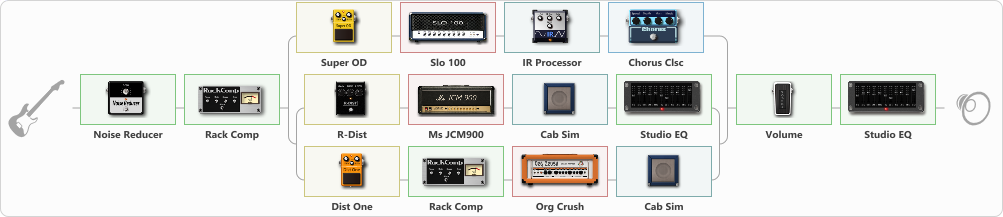# Black Album Tone (Mid scooped)

Discussion in 'ToneLib-GFX presets' started by clever1, Jun 15, 2021.

1. Black Album Tone (Mid scooped)

Preset name: Black Album mid scoop

This is preset is the Mid scooped version of the Black album tone. If you are recording, these should be atleast 2 tracks panned to 75 Right/ 75 Left and a little higher than the Mid present guitars. You can also record 2 more tracks on 15 Right / 15 Left Pan to give it a more present tone.

Related video:

Effects chain:Effect: "Noise Reducer" (Dynamics / Filter), active - "yes"
{
"Sens" = 75
"Mode" = Soft
}

Effect: "Rack Comp" (Dynamics / Filter), active - "yes"
{
"Threshold (dB)" = -32
"Ratio" = 8
"Attack" = Fast
"Release (ms)" = 86
"Knee" = 37
"Level (dB)" = 0
}

Effect: "Splitter" (Dynamics / Filter)
{
"A-Bypass" = Off
"A-Pan" = 31
"A-Level" = 58
"B-Bypass" = Off
"B-Pan" = -33
"B-Level" = 49

'A' branch:
{

Effect: "Super OD" (Overdrive / Distortion), active - "yes"
{
"Drive" = 70
"Tone" = 66
"Level" = 91
}

Effect: "Slo 100" (Amp simulators), active - "yes"
{
"Gain" = 56
"Bass" = 84
"Middle" = 30
"Treble" = 60
"Presence" = 75
"Master" = 68
"Level (dB)" = 2
}

Effect: "IR Processor" (Cabinets), active - "yes"
{
"IR" = Black_L
"Low Cut (Hz)" = 0
"Hi Cut (kHz)" = 20.0
"Mix" = 100
"Level (dB)" = 0
}

Effect: "Chorus Clsc" (Modulation / Sfx), active - "yes"
{
"Speed" = 2.0
"Depth" = 47
"Center" = 1.7
"Mode" = Stereo
}
}
'B' branch:
{

Effect: "Splitter" (Dynamics / Filter)
{
"A-Bypass" = Off
"A-Pan" = -50
"A-Level" = 59
"B-Bypass" = Off
"B-Pan" = 62
"B-Level" = 51

'A' branch:
{

Effect: "R-Dist" (Overdrive / Distortion), active - "yes"
{
"Drive" = 64
"Tone" = 55
"Level" = 50
}

Effect: "Ms JCM900" (Amp simulators), active - "yes"
{
"Gain" = 69
"Bass" = 75
"Middle" = 22
"Treble" = 60
"Presence" = 62
"Master" = 73
"Level (dB)" = 0
}

Effect: "Cab Sim" (Cabinets), active - "yes"
{
"Model" = 4x12" 1960 T75
"Level (dB)" = 0
}

Effect: "Studio EQ" (Dynamics / Filter), active - "yes"
{
"31 Hz" = 0
"62 Hz" = -2
"125 Hz" = 0
"250 Hz" = -3
"500 Hz" = -5
"1 kHz" = -7
"2 kHz" = -4
"4 kHz" = -5
"8 kHz" = -3
"16 kHz" = 0
"Level (dB)" = 0
}
}
'B' branch:
{

Effect: "Dist One" (Overdrive / Distortion), active - "yes"
{
"Drive" = 42
"Tone" = 59
"Level" = 37
}

Effect: "Rack Comp" (Dynamics / Filter), active - "yes"
{
"Threshold (dB)" = -20
"Ratio" = 5
"Attack" = Fast
"Release (ms)" = 250
"Knee" = 25
"Level (dB)" = 0
}

Effect: "Org Crush" (Amp simulators), active - "yes"
{
"Gain" = 69
"Bass" = 69
"Middle" = 16
"Treble" = 36
"Presence" = 66
"Master" = 65
"Level (dB)" = 0
}

Effect: "Cab Sim" (Cabinets), active - "yes"
{
"Model" = 4x12" Cali v30
"Level (dB)" = 0
}
}
}
}
}

Effect: "Volume" (Dynamics / Filter), active - "yes"
{
"Pedal Pos." = 70
"Pan" = 0
}

Effect: "Studio EQ" (Dynamics / Filter), active - "yes"
{
"31 Hz" = 3
"62 Hz" = 2
"125 Hz" = 3
"250 Hz" = -3
"500 Hz" = -6
"1 kHz" = -5
"2 kHz" = -4
"4 kHz" = 2
"8 kHz" = 3
"16 kHz" = 2
"Level (dB)" = 0
}

Note: You will need to download and install the ToneLib-GFX software to use the preset.

#### Attached Files:

• ###### Black_Album_Tone_Mid_scooped.tlgfx
File size:
2.5 KB
Views:
5,705
iiTsGiga, umirva, Max Hart and 22 others like this.
2. ### Tony the MetalheadActive Member

3. Hello there, here is the IR used in the preset.

File size:
2.1 KB
Views:
25